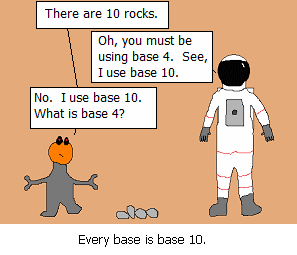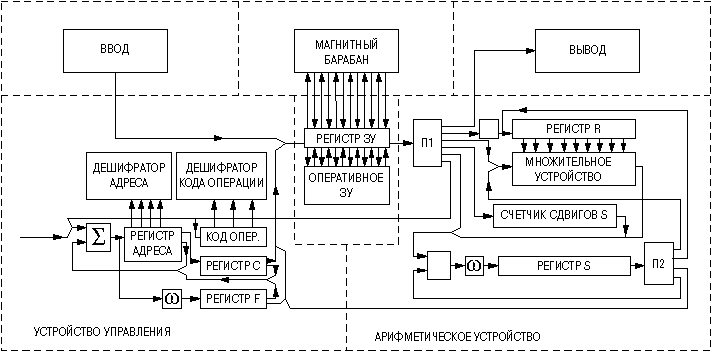随笔：9 文章：0 评论：0 引用：0 精灵轶事 C++ Player

# 2009年8月26日

2009年7月初7
posted @ 2009-08-26 15:53 pterosaur 阅读(149) | 评论 (0)编辑 收藏

# 2009年6月30日

摘要: 登记一个表格,和程序没啥关系,不相关的请忽略.权当冒泡.同轴电缆接头特性:  Connector Name Frequency Range ...  阅读全文
posted @ 2009-06-30 21:07 pterosaur 阅读(149) | 评论 (0)编辑 收藏

# 2009年6月20日

1. [笑话]所有进制都是10进制

1,2,3,4,5,6,7,8,9,10,11,12……

1,2,3,4,5,10,11,12,13,14……2. 生活中的进制系统

1km = 1000m, 1min = 60sec, 1day = 24hour, 1yard = 3ft, 1ft = 12inch

…………………………………………………………

1,10,11,100,101,110,111……

3.不安分的数学家

(bnbn-1bn-2…b3b2b1b0.b-1b-2b-3…)N = k=-…nbkNp  (N为计数的进制，称之为“基”)

N为正整数的时候，就被称为标准N进制系统，为了保证数值的唯一表示N进制系统每一位的数值都不能超过NСетунь 70 结构图，懂俄文的朋友帮忙翻译下了

(1234567890)10 = (2846648290)-10

(12231)2i = (9-10i)10

a)       通过交换1-1，就可以得到一个数的相反数

b)       一个数的正负号由他的最高位的非零的数给出

c)       四舍五入运算被简化成了截断运算

posted @ 2009-06-20 21:03 pterosaur 阅读(316) | 评论 (0)编辑 收藏

# 2009年6月19日

[转自http://yueweitang.org/blog/posts/inverse-square-root-algorithm-analysis.html]float InvSqrt(float x){float xhalf = 0.5f * x;int i = *(int*)&x;i
= 0x5f3759df - (i >> 1);x
= *(float*)&i;x
= x * (1.5f - xhalf * x * x);return x;}$x=1.M_x 2^{E_x-127}$$I_x = 2^{23}E_x+M_x$

int i = *(int*)&x; 这条语句把$x$转成$i=I_x$

i = 0×5f3759df - (i>>1); 这条语句从$I_x$计算$I_{1/\sqrt{x}}$

y = *(float*)&i; 这条语句将$I_{1/\sqrt{x}}$转换为$1/\sqrt{x}$

y = y*(1.5f - xhalf*y*y); 这时候的y是近似解；此步就是经典的牛顿迭代法。迭代次数越多越准确。$y=1/\sqrt{x}$，用$x=(1+m_x)2^{e_x}$$y=(1+m_y)2^{e_y}$带入之后两边取对数，再利用近似表示$\log_2(1+z)\sim z+\delta$，算一算就得到$I_y = \frac{2}{3}(127-\delta)2^{23}-I_x/2$

2003年Chris Lomont还写了一篇文章对这些代码进行了分析，有兴趣的读者可以下载阅读。

posted @ 2009-06-19 17:16 pterosaur 阅读(453) | 评论 (0)编辑 收藏

# 2009年6月6日

明天开始今年的第三次北京之行

1＃include <stdio.h>
2int main(){
3printf("一帆风顺!\n");
4}
posted @ 2009-06-06 20:48 pterosaur 阅读(140) | 评论 (0)编辑 收藏

# 2009年6月1日

1def qsort():
2c = lambda f, v1, v2 : \
3([] if len(v2) == 0 else v2[0:1+ f(f, v1, v2[1:len(v2)])) if len(v1)==0 \
4else v1[0:1]+f(f,v1[1:len(v1)],v2) if len(v2) == 0 \
5else v1[0:1]+f(f,v1[1:len(v1)],v2) if v1<v2 \
6else v2[0:1]+f(f,v1,v2[1:len(v2)]);
7q = lambda f, c, arr :\
8arr if len(arr) < 2 else \
9c(c, f(f, c, arr[0:int(len(arr)/2)]), f(f, c, arr[int(len(arr)/2):len(arr)]));
10return lambda arr : q(q,c,arr);
11posted @ 2009-06-01 15:22 pterosaur 阅读(495) | 评论 (0)编辑 收藏posted @ 2009-06-01 11:38 pterosaur 阅读(207) | 评论 (0)编辑 收藏

# 2009年5月31日

（该题目可以说是骨灰级的题目了，我做了简单的改变，便于分析）int main(){int exist = 0;int x = 0;printf(scanf(
"%d"&x);for(int n = 1; n * n < x * 2++ n){int a = (2 * x / n - n + 1/ 2;if( n * a + n * (n - 1/ 2 == x){for(int i = 0; i < n; ++i)printf(
"%d ", a + i);printf(
"\n");have
= 1;}}if(!exist)printf(
"none\n");}1, 1, 2, 1, 2, 2, 2, 1, 3, 2, 2, 2, 2, 2, 4, 1, 2, 3, 2, 2

1. Number of odd divisors of n;
2. Number of ways to write n as difference of two triangular numbers;
3. Number of ways to arrange n identical objects in a trapezoid;
4. Number of sums of sequences of consecutive positive integers including sequences of length 1;
5. Number of factors in the factorization of the Chebyshev polynomial of thee first kind T_n(x);
6. Number of ways to present n as sum of consecutive integers;
7. Number of factors in the factorization of the polynomial x^n+1 over the integers.

posted @ 2009-05-31 19:55 pterosaur 阅读(654) | 评论 (0)编辑 收藏

# 2009年5月30日

posted @ 2009-05-30 16:08 pterosaur 阅读(132) | 评论 (0)编辑 收藏
CALENDER
 < 2023年2月 >
2930311234
567891011
12131415161718
19202122232425
2627281234
567891011

•